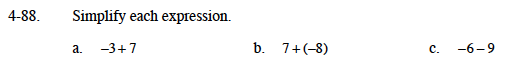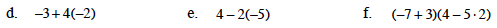### Home > MC1 > Chapter 4 > Lesson 4.3.1 > Problem4-88

4-88.For parts (a) and (b), remember that adding negative numbers is like taking steps backwards on a tightrope. Does it help to draw a diagram?

One method for subtracting integers is to make a diagram of the first integer using positive and negative tiles. Then remove the second integer from the diagram. If there are not enough tiles, add zero pairs.Parts (d) and (e) both have terms which need to be simplified before they can be combined with the other
term in the expression.

The answer for part (d) is −11.

Simplify inside the parentheses.
(−4)(4 − 10)
(−4)(−6)

Multiply.
(−4)(−6) = 24

14

Use the hints provided for parts (a),(b), and (c) for help with adding and subtracting.

24